# ISEE Middle Level Math : Median

## Example Questions

### Example Question #31 : How To Find Median

Find the median in this set of numbers: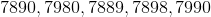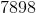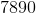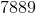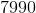Explanation:

First, order the numbers from least to greatest: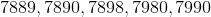Then, identify the middle number:Answer: The median is### Example Question #32 : How To Find Median

Find the median in this set of numbers: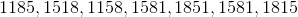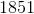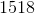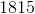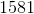Explanation:

First, order the numbers from least to greatest: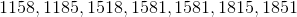Then, identify the middle number in the set:### Example Question #33 : How To Find Median

Find the median in this set of numbers: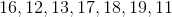Explanation:

First, order the numbers from least to greatest: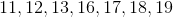Then, identify the middle number:Answer: The median is.

### Example Question #34 : How To Find Median

Find the median in this set of numbers: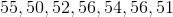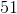Explanation:

First, order the numbers from least to greatest: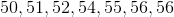Then, find the middle number:Answer: The median is.

### Example Question #31 : How To Find Median

What is the median in this set of numbers: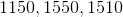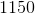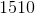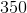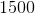Explanation:

Order the numbers from least to greatest: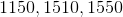Then identify the middle number:### Example Question #36 : How To Find Median

Find the median in this set of numbers: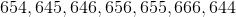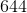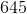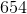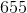Explanation:

First, order the numbers from least to greatest: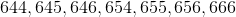Then, identify the middle number:### Example Question #37 : How To Find Median

Find the median of 56, 12, 34, 73, and 66.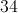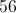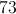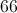Explanation:

First, place the numbers in order from smallest to largest: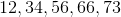Then, identify the middle number, as this is the median.

The median is therefore 56.

### Example Question #38 : How To Find Median

Find the median in this set of numbers: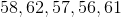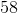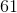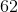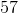Explanation:

First, order the numbers from least to greatest: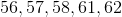Then, identify the middle number:Answer: The median is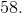### Example Question #39 : How To Find Median

Find the median in this set of numbers: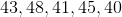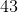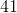Explanation:

First order the numbers from least to greatest: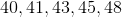Then find the middle number:Answer: The median is.

### Example Question #31 : Median

Find the median in this set of numbers: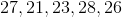Explanation:

First, order the numbers from least to greatest: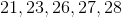Now we can easily identify the middle number:Answer: The median is.

### All ISEE Middle Level Math Resources